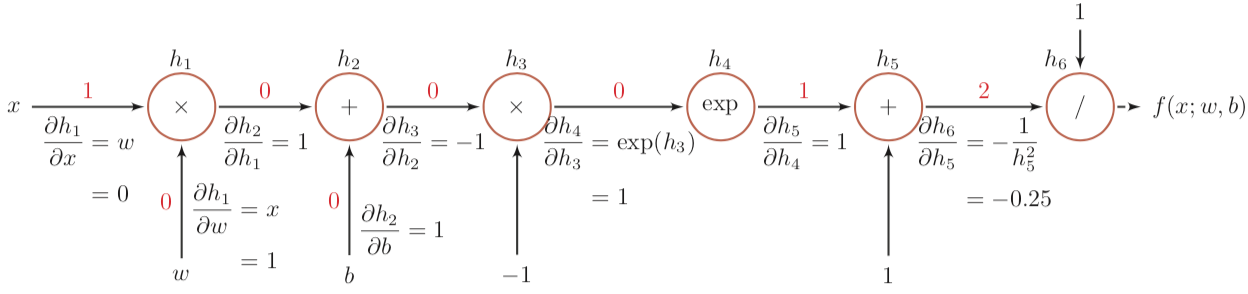# 神经网络中的自动微分与反向传播

• 加法法则：任意两个节点间的梯度为它们两节点之间所有路径的偏微分之和；
• 链式法则：一条路径的偏微分为路径上各相邻节点间偏微分的连乘。

### 一个例子$$\frac{\partial{f(x; w, b)}}{\partial{h_2}} = \frac{\partial{f(x; w, b)}}{\partial{h_6}} \cdot \frac{\partial{h_6}}{\partial{h_5}} \cdot \frac{\partial{h_5}}{\partial{h_4}} \cdot \frac{\partial{h_4}}{\partial{h_3}} \cdot \frac{\partial{h_3}}{\partial{h_2}} = 1 \times -0.25 \times 1 \times 1 \times -1 = 0.25$$ $$\frac{\partial{f(x; w, b)}}{\partial{b}} = \frac{\partial{f(x; w, b)}}{\partial{h_2}} \cdot \frac{\partial{h_2}}{\partial{b}} = 0.25 \times 1 = 0.25$$ $$\frac{\partial{f(x; w, b)}}{\partial{x}} = \frac{\partial{f(x; w, b)}}{\partial{h_2}} \cdot \frac{\partial{h_2}}{\partial{h_1}} \cdot \frac{\partial{h_1}}{\partial{x}} = 0.25 \times 1 \times 0 = 0$$

## 全连接神经网络 FNN

digraph {
label="全连接网络的计算图";
rankdir=LR;
node [shape=none];
x; w[label="W_i"]; b[label="b_i"]; y1 [label="y'"]; y; e [label="Δ"];
node [shape=circle];
{ rank=same; y -> L; }
subgraph cluster_hidden_Layers {
label="隐藏层 ×N";
h1 [label="x"];
h2 [label="+"];
h3 [label="σ"];
{ rank=same; w -> h1; }
{ rank=same; b -> h2; }
}
x -> h1 -> h2 -> h3 -> y1 -> L -> e;
}


## 卷积神经网络 CNN

### 卷积层（Convolution Layer）

$$\frac{\partial{f(Y)}}{\partial{W}} = \frac{\partial{f(Y)}}{\partial{Y}} \otimes X$$ $$\frac{\partial{f(Y)}}{\partial{X}} = \frac{\partial{f(Y)}}{\partial{Y}} \tilde{\otimes} W$$

digraph {
label="卷积运算";
rankdir=LR;
conv [label="⊗", shape=circle];
node [shape=none];
"输入 X" -> conv -> "输出 Y";
{ rank=same; "卷积核 W" -> conv; }
}


### 池化层（Pooling Layer）

• 最大池化（Max Pooling）：上层节点仅对池化区域内的最大节点有梯度 1，对其它节点的梯度为 0；
• 平均池化（Mean Pooling）：假设池化面积为 n，则上层节点对每个元素的梯度为 1/n。

Max 运算的导数并不连续，所以这里的梯度只能算是一种近似。

## 循环神经网络 RNN

digraph {
label="循环神经网络的计算图";
node [shape=none];
x1; w1[label="W"]; b1[label="b"]; h1;
x2; w2[label="W"]; b2[label="b"]; u2[label="U"]; h2;
u3[label="U"]; etc[label="..."];
node [shape=circle];
node [label="×"]; op11; op20; op21; op30;
node [label="+"]; op12; op22[style=bold, xlabel="z2"];
node [label="σ"]; op13; op23;
{
rank=same;
x1 -> op11;
op11 -> op12 -> op13 [arrowhead=normal, style=bold, color=red];
op13 -> h1;
}
op11 -> w1 [dir=back, arrowhead=normal, style=bold, color=red];
op12 -> b1 [dir=back];
op13 -> op20 [arrowhead=normal, style=bold, color=red];
{ rank=same; op20 -> u2 [dir=back]; }
op20 -> op22 [arrowhead=normal, style=bold, color=red];
{
rank=same;
x2 -> op21;
op21 -> op22 -> op23 -> h2 [arrowhead=normal, style=bold, color=red];
}
op21 -> w2 [dir=back, arrowhead=normal, style=bold, color=red];
op22 -> b2 [dir=back];
op23 -> op30;
{ rank=same; op30 -> u3 [dir=back]; }
op30 -> etc;
}


$h_1, h_2, \cdots, h_n$ 只是个中间结果，可以经过其它可微变换输出 $y$ 然后接损失函数得到误差，这一部分跟 FNN 是一样的所以省略了。